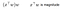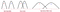# Complete Feature Selection Techniques 4 - 3 Dimension Reduction

Summarize math intuition and demonstrate PCA, LDA, MDS, ISOMAP, T-SNE, UMAP for feature dimension reduction

Complete Feature Selection Techniques

1. Statistical Test & Analysis
2. Correlation Analysis
3. Dimension Reduction
4. Model Driven

# PCA and LDA

Both Principal Component Analysis(PCA) and Linear Discriminant Analysis(LDA) are linear transformation techniques.

Linear transformation can scale and rotate data points from original axis(a.k.a space) into a new axis, and the data distribution will be changed accordingly during the scaling and rotating.

So what PCA and LDA do, is under a certain criteria to find the best transformation(scale and rotate).

## Linear Transformation and Orthogonal Projection

The following is an example of Linear Transformation and Orthogonal Projection.

x is any data point vector in training set X(x1, x2, , , xn) X is d x n, and assume X is mean value centered.

w is any vector in orthogonal transformation matrix W(w1, w2, , , wd) W is d x d and ∥wᵢ∥ = 1

Then orthogonal project x on w is as below, and the project value is x new value on axis wx projection on w

## PCA Transformation Object

PCA object is to find the transformation which can maximize the whole dataset variance.

Based on the variance and orthogonal project definition, for a given new axis w, the variance of transformed training set is

Because the training set X is mean centered, x bar is 0

The ultimate PCA object function is as below, Cₙ is training set X covariance matrix. (Assume X is mean centered)

Resolve the optimization function with Lagrangian multiplier (condition on ∥wᵢ∥ = 1), we can get maximization condition is

So PCA transformation matrix W is eigenvectors of training data(centered) covariance matrix.

Because eigenvectors provide perfect variance partition, the total transformed dataset variance is partitioned by each eigenvector, so we can reduce some relatively low variance(a.k.a less informative) eigenvectors, and this can be used for feature dimension reduction.

## LDA Transformation Object

LDA object is to find the transformation which can maximize class separability in the dataset

To maximize class separability, LDA try to find a transformation to achieve

1. Separate each class center(a.k.a class mean) as far as possible
2. Scale each class range as small as possible, so there is less overlap between different classesIntuition for separability

The separation of each class center can be measured by the variance of mean values of all classes

Suppose training set X has n classes, μ is total mean, μᵢ is mean for class i

For a given new axis w, the class center variance is

Because the training set X is mean centered, μ is 0, so

The short expression for class center variance value is below, and LDA expects transformation w enlarge the value.

LDA also requires transformation w reduces the sum of variance of each class.

The variance sum of all class is

And the short expression is as below, and LDA expects transformation w reduce the value.

Ultimately, LDA put the above two requirements together and looking for the transformation w to maximize the below function

Resolve the optimization, maximization condition is

So LDA transformation matrix W is eigenvectors as well, so like PCA we can reduce some relatively small eigenvalue eigenvectors to implement feature dimension reduction.

## PCA and LDA Python Example

`import matplotlib.pyplot as pltfrom sklearn import datasetsfrom sklearn.decomposition import PCAfrom sklearn.discriminant_analysis import LinearDiscriminantAnalysis%matplotlib inlineiris = datasets.load_iris()X = iris.datay = iris.targettarget_names = iris.target_namespca = PCA(n_components=2) # Keep first 2 PCX_r = pca.fit(X).transform(X)lda = LinearDiscriminantAnalysis(n_components=2)# Keep first 2 PCX_r2 = lda.fit(X, y).transform(X)colors = ['navy', 'turquoise', 'darkorange']for color, i, target_name in zip(colors, [0, 1, 2], target_names):    plt.scatter(X_r[y == i, 0],                 X_r[y == i, 1],                 color=color,                 label=target_name)plt.legend(loc='best', shadow=False, scatterpoints=1)plt.title('PCA of IRIS dataset')plt.figure()for color, i, target_name in zip(colors, [0, 1, 2], target_names):    plt.scatter(X_r2[y == i, 0],                 X_r2[y == i, 1],                 color=color,                label=target_name)plt.legend(loc='best', shadow=False, scatterpoints=1)plt.title('LDA of IRIS dataset')`

LDA has better separability

# MDS and ISOMAP

Multidimensional Scaling(MDS) and Isometric Feature Mapping(ISOMAP) are two very similar non-linear dimension reduction techniques.

The feature of MDS and ISOMAP is

In the dimension reduction process, both of them will target to preserve pair distances for all data points.

It means, MDS and ISOMAP try to find the lower dimensions in which any two data points have same distance as in high dimensions.

## The common process for MDS and ISOMAP is as below:

Assume training set X has N instances and each instance has D dimensions

1. Construct distance matrix D(N x N), cell Dᵢⱼ represents pair distance of training data point i and j
2. Define matrix Y, Y is (N x K) and K< D
3. Assume training set X is mapped to Y, because the mapping keeps instance pairwise distance, so ∥Yᵢ - Yⱼ∥² = Dᵢⱼ²
4. Define an inner product matrix G = YYᵗ, and computer G (Please check reference 6,7,8 )
5. Because G is symmetric matrix, its Eigendecomposition can be

7. Because rank(G) = rank(YYᵗ) = rank(Y) = K, so G only has K non-zero eigenvalues, remove zero eigenvalues and corresponding eigenvectors

8. From G = YYᵗ, the lower dimension(K<N) data points Y (N x K) is as below

## Difference Between MDS and ISOMAP

The above process is common for both MDS and ISOMAP, the only difference is, MDS and ISOMAP may use different way to define and calculate distance.

MDS can use Euclidean, Manhattan, Cosine or Custom Distance. The above resolution process is based on Euclidean distance, and for other distance we need construct a object function(e.g. the distance difference between high and low dimensions) and use gradient descend to resolve the lower dimension mapping.

ISOMAP use geodesic distance, it use neighborhood graph connection to find the shortest path between two data points, and use the shortest path as distance(Please check reference 9,11)

## MDS and ISOMAP Python Example

MDS vs PCA

`from sklearn.manifold import MDSmds = MDS( n_components=2, metric=True) # Keep first 2 PCmds_r = mds.fit_transform(X)plt.figure()for color, i, target_name in zip(colors, [0, 1, 2], target_names):    plt.scatter(mds_r[y == i, 0],                 mds_r[y == i, 1],                 color=color,                label=target_name)plt.legend(loc='best', shadow=False, scatterpoints=1)plt.title('MDS of IRIS dataset')`

ISOMAP

`from sklearn.manifold import Isomapfig, ax = plt.subplots(1,3,figsize=(15, 5)) for idx, neighbor in enumerate([2, 20, 100]):     isomap = Isomap( n_components=2, n_neighbors=neighbor)    new_X_isomap = isomap.fit_transform(X)    ax[idx].scatter(        new_X_isomap[:,0],         new_X_isomap[:,1],         c=y)    ax[idx].set_title("Isomap (n_neighbors=%d)"%neighbor)plt.show()`

# T-SNE and UMAP

From a different perspective, T-SNE and UMAP introduce probability distribution to describe data points relative location and approximate low dimension distribution to high dimension distribution to preserve data points local and global relative positions in dimension reduction.

Normally we only use T-SNE and UMAP to visualize high-dimension data in low-dimension graph.

## General Idea and Process of T-SNE and UMAP

1. For each original high dimension data instance x, construct conditional probability distribution p (Any Other Instance|Current Data Instance) based on the pair distance, closer distances will get higher probability
2. Assume y is the data instance x mapping in low dimension space, and the low dimension conditional probability distribution q satisfies p(xⱼ|xᵢ) = q(xⱼ|xᵢ)
3. Approximate the low dimension distribution to high dimension distribution, and compute the low dimension via a cost function and gradient descend to find the best low dimension matrix y.

## Brief Definition Summary

T-SNE uses Gaussian distribution for High-D distance mapping, t-distribution for Low-D distance mapping, cost function is KL_Divergence.

UMAP use custom distributions for both High-D and Low-D and use Binary Cross Entropy as cost function.

In both T-SNE and UMAP, σᵢ is perplexity which decide the neighborhood size when calculate distance probability, please check reference 14,17 for more details of T-SNE and UMAP parameters.

## T-SNE & UMAP Python Example

`#pip install umap-learnimport umapimport numpy as npfrom sklearn.manifold import TSNEfrom sklearn import datasetsdigits = datasets.load_digits()target_ids = range(len(digits.target_names))tsne = TSNE(n_components=2,             perplexity=50,             random_state=0)tsne_r = tsne.fit_transform(digits.data)umap_ = umap.UMAP(n_components=2,                   n_neighbors= 50,                   random_state=42)umap_r = umap_.fit_transform(digits.data)fig, ax_array = plt.subplots(20, 20)axes = ax_array.flatten()for i, ax in enumerate(axes):    ax.imshow(digits.images[i], cmap='gray_r')plt.setp(axes, xticks=[], yticks=[], frame_on=False)plt.tight_layout(h_pad=0.5, w_pad=0.01)plt.show()plt.scatter(tsne_r[:, 0],             tsne_r[:, 1],             c=digits.target,             cmap='Spectral',             s=5)plt.gca().set_aspect('equal', 'datalim')plt.colorbar(boundaries=np.arange(11)-0.5).set_ticks(np.arange(10))plt.title('T-SNE projection of the Digits dataset')plt.figure()plt.scatter(umap_r[:, 0],             umap_r[:, 1],             c=digits.target,             cmap='Spectral',             s=5)plt.gca().set_aspect('equal', 'datalim')plt.colorbar(boundaries=np.arange(11)-0.5).set_ticks(np.arange(10))plt.title('UMAP projection of the Digits dataset')`

# REFERENCE

Data Scientist & Engineer from Sydney

## More from Summer Hu

Data Scientist & Engineer from Sydney